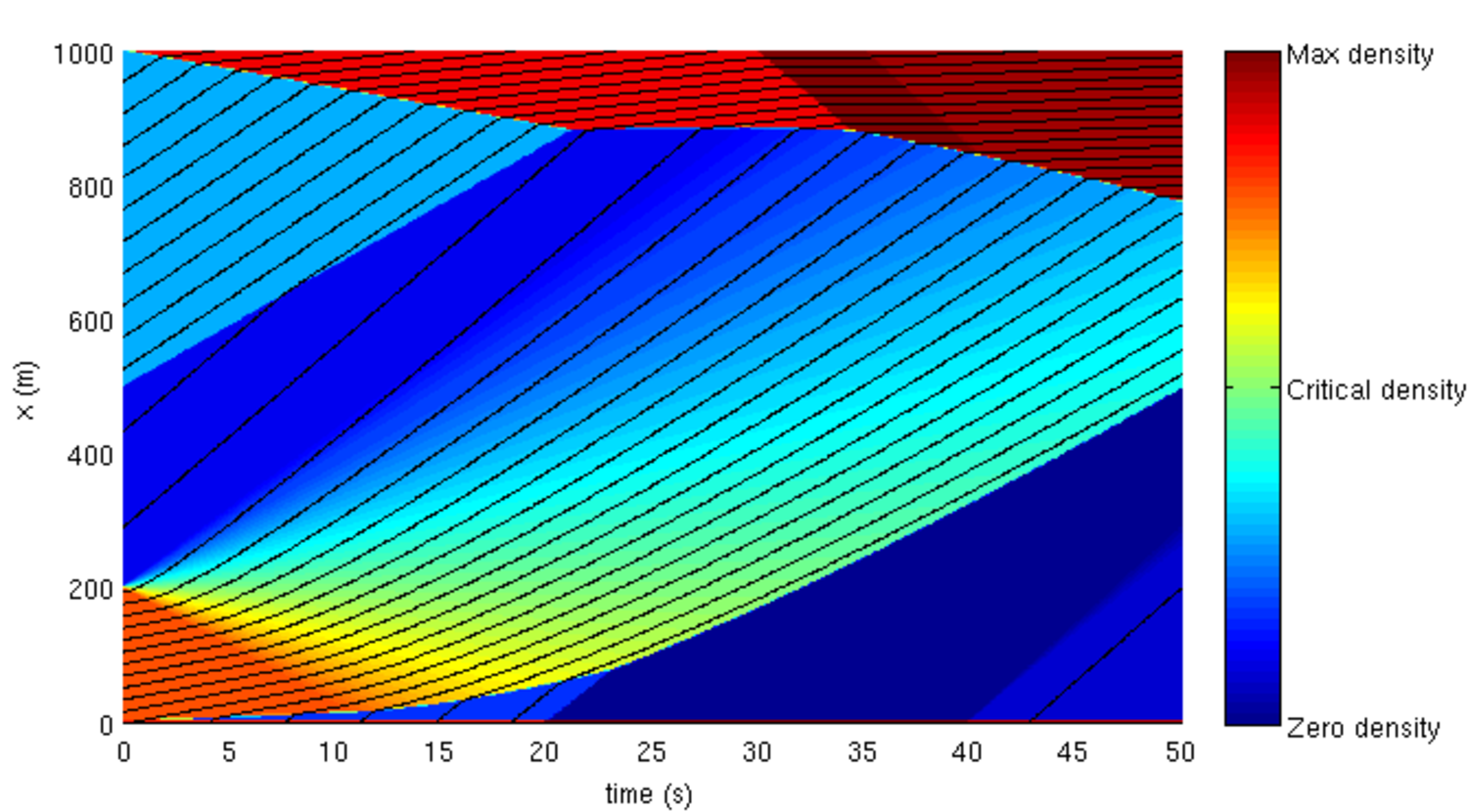# Implementation of an LWR Solver

LWR solver

The Lighthill-Whitham-Richards Partial Differential Equation (LWR PDE) is a seminal equation in traffic flow theory. It leads to common yet widely used traffic flow models for highways. This package proposes a sample implementation for a LWR solver using a new Lax-Hopf method. In the literature, the LWR PDE is typically solved using the Cell Transmission Model (CTM), a Godunov scheme, which requires a grid to compute the solution numerically, and induces specific approximations of the solution (in addition to the errors of the numerical computation).

The proposed approach has the following features:

• It is greed free: in order to compute the value at a given point x and a given time t, it is not necessary to grid space and time (only to prescribe initial and boundary data, and to enter the point where the solution should be computed).
• It is exact: the program presented here computes the solution by evaluating a function numerically (rather than approximating derivatives of the PDE by finite differences).
• It is has small computational cost: since it is not needed to grid the space, the computation is much faster than with finite difference methods (Godunov, CTM, etc.)### 30-second how-to

• script.m contains a sample script which creates a Lax-Hopf solver for a given Greenshields fundamental diagram and solves the Cauchy problem associated with an arbitrary set of initial densities, upstream and downstream flows;
• two functions allow you to plot your results: LH_plot3D and LH_plot2D;
• for advanced users, it is possible to solve the Cauchy problem associated with any concave fundamental diagram. Refer to the manual (PDF) for more details.

### Important notes

• The Matlab functions require Matlab version R2008A or newer. They may work under earlier Matlab versions, but have not been tested.
• No additional toolboxes are required.